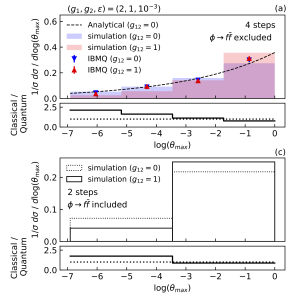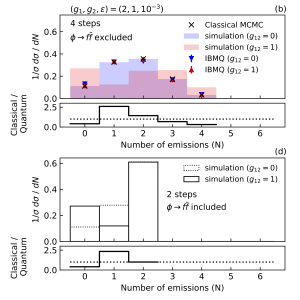# Quantum computing for HEP

## Quantum computing for High Energy Physics

Our QC activities span HEP phenomenology, formal theory, and experiment. In phenomenology, we aim to develop quantum algorithms to enable calculations and simulations that are intractable using classical computers. In formal theory we will develop a new quantum circuit on near term devices that can teleport multiple qubits using fast-scrambling dynamics of black holes, as well as study of the ground state. In experimental HEP we will study how quantum algorithms can improve particle tracking algorithms.

Our HEP QC effort reaches beyond the LBNL Physics Division. We have formed interdisciplinary collaborations between the Physics and Computing Research Divisions, between scientists in HEP and Quantum Chemistry,and between theorists developing algorithms and experimentalists building QC devices. The relationships between HEP theory and QC hardware development are an integral part.

## Simulation of events in high energy collisions

A central HEP question is whether nature at the highest available energies is still described well by the standard model (SM) of particle physics, or if physics beyond the SM (BSM) is required. Connecting experiments to theory requires detailed calculations that are directly comparable to the measurements. In almost all cases, these calculations can only be performed in certain limits of the theory, giving rise to theoretical uncertainties. As measurement precision increases, these uncertainties start to dominate. In particular, for events with high multiplicity of final state particles, the known theoretical algorithms severely limit the accuracy with which predictions can be made. In many cases these limitations are not fundamental, but due to calculation complexity growing exponentially with the number of final state particles. Given that quantum algorithms have been shown to provide exponential speedup over classical calculations in many cases, a focus our research has been to study how quantum algorithms can be used to simulate high multiplicity events without exponential growth.Normalized differential cross section for the emission angle. The top plots show results for the case where boson splitting is neglected, while the bottom plots show the full simulation. One can clearly see the effect of the interference.Normalized differential cross section for the emission angle. The top plots show results for the case where boson splitting is neglected, while the bottom plots show the full simulation. One can clearly see the effect of the interference.

We have shown in simplified models that effects intractable using known classical algorithms can be simulated on quantum computers with only polynomial scaling with particle multiplicity, rather than exponential.The particular simplified model consisted of two types of fermions interacting with bosons, including a “flavor mixing” coupling between them. This flavor mixing gives rise to numerically important interference effects, and the number of amplitudes that can interfere grows exponentially with the number of final state fermions. Standard parton shower algorithms would simply miss these interference effects, while classical algorithms that include them scale exponentially with the number of final states. Our quantum algorithm that explicitly includes all interference effects, yet scales only polynomially in the number of final state fermions. A comparison of the complexity of the quantum and classical algorithms revealed that the quantum algorithm outperforms the classical one for more than 10 fermions in the final state. While currently available quantum hardware is not yet able to run the full parton shower, we were able to run a simplified version to show that the quantum algorithm can clearly pick up the interference effects

The goal going forward is to simulate the dynamics of an effective field theory that can describe long distance radiation of particles in high energy collisions, such as the LHC. We are developing a code library that allows for the simulation of such field theories in very simple setups. In particular, we have been studying one of the important steps in the Jordan, Lee and Preskill algorithm, namely the adiabatic evolution required in the state preparation of the interacting field theory, and are determining the best strategies to perform these steps on NISQ-era devices. Given that reduction of operation errors is critical to quantum computation in the NISQ era, we have also started work on readout error reduction.

## Recovery of scrambled quantum information

Besides directly testing nature at the smallest distance scales, understanding the general behavior of quantum information is a fundamental problem in physics. An important question, originally motivated by the black hole information paradox, is under what conditions can quantum information be recovered after being scrambled? While general relativity predicts that information is lost forever in a black hole, the evolution according to quantum mechanics is unitary, hence reversible, suggesting that there may in principle be a way to recover the information. The standard formulation of this problem is, if a secret quantum state is thrown into a black hole, can it be reconstructed by collecting the Hawking radiation emitted at a later time? Explicit protocols have been developed that allow for the decoding of any scrambling quantum system and, in effect, teleporting a quantum state from one copy of the system to another.

A key result of the research on quantum information scrambling was an experimental implementation of the Yoshida-Kitaev protocol, proving that it can explicitly distinguish between decoherence and chaotic scrambling dynamics. The protocol was implemented in both a trapped ion qubit system and a superconducting qutrit system. While these results open the door to experimentally measuring quantum scrambling with built-in verifiability, a number of key questions remain. In particular, although the Yoshida-Kitaev protocol can be efficiently implemented to teleport a single qubit, it requires an exponentially greater computational complexity to teleport multiple qubits. To this end, we propose to develop and explore a novel protocol which overcomes this exponential complexity.

## Quantum Ternary Logic for field theory simulations

The study of quantum information propagation has provided valuable insights that reach beyondthe field of quantum computation. For example, scrambling, the rapid spread of quantum information in a strongly interacting systems, connects diverse phenomena from black hole physics to the transport in exotic non-Fermi liquids. We aim to develop quantum devices that can track the flow of quantum information through all degrees of freedom in a controlled setting and thereby may shed light on fundamental questions in quantum cosmology and condensed matter physics.

Recently we demonstrated genuine scrambling between two “qutrits” (three energy level analogs of qubits) implemented in a transmon-based digital quantum processor. Owing to the fast repetition rates of experiments with superconducting circuits, we were able to do full process tomography of the scrambling unitary, monitoring exactly how initially localized operators spread when they are being scrambled. This scrambling unitary is the crucial building block of a five-qutrit quantum circuit which can verify that the spread of quantum information is the effect of scrambling and not decoherence (which also can manifest itself as the loss of local coherence). This circuit enables the work in Sec.~\ref{sec:blackholes}, as has an alternative interpretation in the black hole information paradox where information, apparently lost (scrambled) in a black hole, is recovered through a teleportation protocol. We demonstrated average recovery fidelity of 54%, well above the expected classical fidelity of 33\% thus demonstrating a clear quantum advantage.

The pivotal technological advance was the development of the qutrit, which significantly reduced the number of required gates relative to using qubits. In the NISQ era, where the number of entangling operations is typically the limiting factor in processor performance, the use of ternary quantum logic can significantly expand the computational power of a given device~\cite{DBLP:conf/isca/2019,kiktenko2019scalable}.

## Quantum Algorithms for Pattern Recognition

Under current funding we have demonstrated in the HEP.QPR project how to express the HEP particle tracking problem as a binary optimization problem that can be solved by a D-Wave quantum annealer (QA), and potentially by other quantum optimization algorithms like Variational Quantum-Eigensolvers and Quantum Assisted Optimization Algorithms. HEP.QPR prototypes showed that the QA physics performance is competitive with classical track seeding algorithms at luminosities of the current LHC. Encouraged by these early findings we want to further explore three directions

1. Optimizing QUBO performance on next-generation Quantum Annealers
2. Comparing Digital Annealers to Quantum Annealers
3. Global Tracking Optimization in Dense Jets